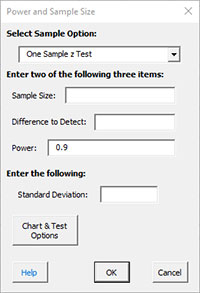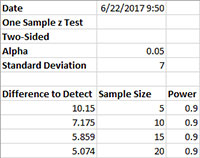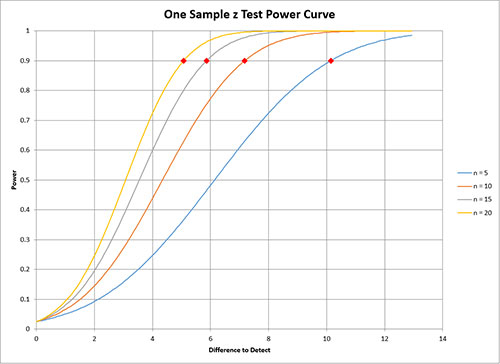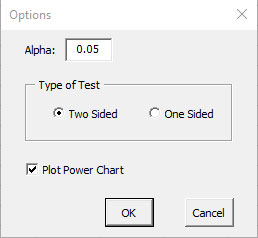# Power and Sample Size HelpSPC for Excel will calculate the power, sample size and difference to detect for the one sample z test, the one and two sample t test and the one and two proportion test.  This describes how to do this for the one sample z test.  The other tests are very similar.

1. Select “Sample Tests” from the “Statistical Tools” panel in the SPC for Excel ribbon

2. Select the “Power and Sample Size” option from the “Sample Tests” form.  Select OK and the input form below is shown.3. Select the sample option you want.  In this example, we are using One Sample z Test.  When you select the sample, the form is updated for the option for that sample.Enter two of the following three items:

• Sample Size
• Difference to Detect
• Power (default is 0.9)

You many enter multiple values.  For example, you could enter 5, 10, 15, 20 for sample sizes.  Separate the multiple entries by a comma.

You must enter a value for the standard deviation.

4.  After entering the values, select OK.  For example, suppose you entered differences to detect of 5, 8, 10 with a standard deviation of 7.  The results on summarized on a worksheet called “Power SPC for Excel.”  This output is shown below.  The sample sizes required for power = .9 were calculated.  The actual power is also given.If the option to generate the power chart was true, the power chart is generated.  An example is shown below.5. The “Chart & Test Options” options are shown below.  You may change the defaults if needed.• Alpha: confidence level; default = 0.05
• Type of Test:
• Two sided (default)
• One sided
• Plot Power Chart: default is true